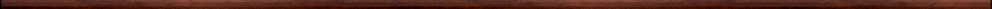Maple worksheets on approximation of functionsNumerical methods topics:

They are all compatible with Classic Worksheet Maple 10.The method of moments - moment.mws

• An introduction to the moment scheme for constructing approximating polynomials
• The general moment scheme
• A procedure for constructing moment polynomials - momentpoly

Local Taylor series approximation of functions - loctaylor.mws

• Defining procedures and viewing Maple library code
• Local Taylor series approximation for functions
• A procedure for constructing local Taylor series approximations - loctaylor

Chebyshev polynomials and Chebyshev series - chebfit.mws

• Definition of Chebyshev polynomials
• Properties of Chebyshev polynomials - orthogonality relations
• Expressing a polynomial as a Chebyshev sum
• An alternative method for calculating Chebyshev coefficients
• Chebyshev series
• Example: the Chebyshev series for exp(x)
• A procedure for computing Chebyshev polynomial: chebseries

Using interpolating polynomials to approximate functions - interpoly.mws

• A procedure constructing an interpolating polynomial approximation - interpoly
• interpoly: examples with evenly spaced nodes
• Using an interpolating polynomial to emulate a finite Chebyshev series
• interpoly: general examples

Jacobi polynomials and interpolating polynomials - jacobi.mws

• Jacobi polynomials
• Zeros of the Jacobi polynomials
• interpoly: examples with nodes spaced in the pattern of the zeros of Jacobi polynomials

The Remez algorithm for constructing minimax polynomial approximations - minimax.mws

• The minimax polynomial approximation for a continuous function on a closed interval
• The calculation of a minimax polynomial - introduction to the Remez algorithm
• An error estimate for the minimax polynomial
• The calculation of a minimax polynomial for exp(x) on [-1,1]

The Remez algorithm for constructing minimax rational approximations: version I -
using an iterative method for obtaining the minimax error
- ratminmax.mws

• The calculation of a minimax rational approximation for ln(1+x) on [0,1]
• A utility routine for calculating the critical points of a function - critpts
• The calculation of a minimax rational approximation for exp(x) on [-1,1]
• Comparison of polynomial and rational minimax approximations

The Remez algorithm for constructing minimax rational approximations: version II -
solving a rational equation to obtain the minimax error
- ratminmax2.mws

• The calculation of a minimax rational approximation for ln(1+x) on [0,1]
• A utility routine for calculating the critical points of a function - critpts
• The calculation of a minimax rational approximation for exp(x) on [-1,1]
• Comparison of polynomial and rational minimax approximations

A procedure implementing the Remez algorithm - remez.mws

• A procedure for constructing minimax polynomial and rational approximations via the Remez algorithm - remez
• remez: examples

The Remez algorithm: standard and non-standard error curves for rational approximations to an even function - RZeven.mws

• Standard and non-standard error curves
• A minimax rational approximation for cos(Pi/4*x) on [-1,1]
• A minimax rational approximation for cosh(x) on [-1,1]

Using the Remez algorithm for constructing polynomial approximations for sin(x) and cos(x) on [-Pi/4,Pi/4] - RZsincos.mws

More examples of minimax rational approximations - RZexamp.mws

Testing the Remez algorithm with "badly behaved" functions - RZexamp2.mws

Function approximation procedures - fcnapprx.zip

Top of page

Main index